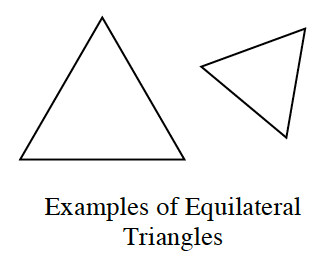### Home > GC > Chapter 1 > Lesson 1.1.2 > Problem1-15

1-15.The shapes at right are examples of equilateral triangles. How can you describe an equilateral triangle? Make at least two statements that seem true for all equilateral triangles. Then trace these equilateral triangles on your paper and draw one more in a different orientation.

What do these triangles have in common?

They have $3$ sides of equal length.
They have $3$ angles of equal measures.